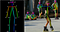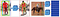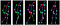# An Overview of Human Pose Estimation with Deep Learning

## An introduction to the techniques used in Human Pose Estimation based on Deep Learning.

Written by Bharath Raj with feedback from Yoni Osin.

A Human Pose Skeleton represents the orientation of a person in a graphical format. Essentially, it is a set of coordinates that can be connected to describe the pose of the person. Each co-ordinate in the skeleton is known as a part (or a joint, or a keypoint). A valid connection between two parts is known as a pair (or a limb). Note that, not all part combinations give rise to valid pairs. A sample human pose skeleton is shown below.Left: COCO keypoint format for human pose skeletons. Right: Rendered human pose skeletons. (Source)

Knowing the orientation of a person opens avenues for several real-life applications, some of which are discussed towards the end of this blog. Several approaches to Human Pose Estimation were introduced over the years. The earliest (and slowest) methods typically estimating the pose of a single person in an image which only had one person to begin with. These methods often identify the individual parts first, followed by forming connections between them to create the pose.

Naturally, these methods are not particularly useful in many real-life scenarios where images contain multiple people.

## Multi-Person Pose Estimation

• The simple approach is to incorporate a person detector first, followed by estimating the parts and then calculating the pose for each person. This method is known as the top-down approach.
• Another approach is to detect all parts in the image (i.e. parts of every person), followed by associating/grouping parts belonging to distinct persons. This method is known as the bottom-up approach.

Typically, the top-down approach is easier to implement than the bottom-up approach as adding a person detector is much simpler than adding associating/grouping algorithms. It is hard to judge which approach has better performance overall as it really comes down to which among the person detector and associating/grouping algorithms is better.

In this blog, we will focus on multi-person human pose estimation using deep learning techniques. In the next section, we will review some of the popular top-down and bottom-up approaches for the same.

# Deep Learning Methods

## 1. OpenPose

As with many bottom-up approaches, OpenPose first detects parts (keypoints) belonging to every person in the image, followed by assigning parts to distinct individuals. Shown below is the architecture of the OpenPose model.

The OpenPose network first extracts features from an image using the first few layers (VGG-19 in the above flowchart). The features are then fed into two parallel branches of convolutional layers. The first branch predicts a set of 18 confidence maps, with each map representing a particular part of the human pose skeleton. The second branch predicts a set of 38 Part Affinity Fields (PAFs) which represents the degree of association between parts.

Successive stages are used to refine the predictions made by each branch. Using the part confidence maps, bipartite graphs are formed between pairs of parts (as shown in the above image). Using the PAF values, weaker links in the bipartite graphs are pruned. Through the above steps, human pose skeletons can be estimated and assigned to every person in the image. For a more thorough explanation of the algorithm, you may refer to their paper and to this blog post.

## 2. DeepCut

1. Produce a set of `D` body part candidates. This set represents all possible locations of body parts for every person in the image. Select a subset of body parts from the above set of body part candidates.
2. Label each selected body part with one of `C` body part classes. The body part classes represent the types of parts, such as “arm”, “leg”, “torso” etc.
3. Partition body parts that belong to the same person.

The above problems were jointly solved by modeling it into an Integer Linear Programming (ILP) problem. It is modeled by considering triples `(x, y, z)` of binary random variables with domains as stated in the images below.

Consider two body part candidates `d` and `d'` from the set of body part candidates `D` and classes `c` and `c'` from the set of classes `C`. The body part candidates were obtained through a Faster RCNN or a Dense CNN. Now, we can develop the following set of statements.

• If `x(d,c) = 1` then it means that body part candidate `d` belongs to class `c`.
• Also, `y(d,d') = 1 `indicates that body part candidates `d` and `d'` belong to the same person.
• They also define `z(d,d’,c,c’) = x(d,c) * x(d’,c’) * y(d,d’)`. If the above value is 1, then it means that body part candidate `d` belongs to class `c`, body part candidate `d'` belongs to class `c'`, and finally body part candidates `d,d’` belong to the same person.

The last statement can be used to partition pose belonging to different people. Clearly, the above statements can be formulated in terms of linear equations as functions of `(x,y,z)`. In this way, the Integer Linear Program (ILP) is set up, and the pose of multiple persons can be estimated. For the exact set of equations and much more detailed analysis, you can check out their paper here.

## 3. RMPE (AlphaPose)Effect of duplicate predictions (left) and low confidence bounding boxes (right). (Source)

To resolve this issue, the authors proposed the usage of Symmetric Spatial Transformer Network (SSTN) to extract a high-quality single person region from an inaccurate bounding box. A Single Person Pose Estimator (SPPE) is used in this extracted region to estimate the human pose skeleton for that person. A Spatial De-Transformer Network (SDTN) is used to remap the estimated human pose back to the original image coordinate system. Finally, a parametric pose Non-Maximum Suppression (NMS) technique is used to handle the issue of redundant pose deductions.

Furthermore, the authors introduce a Pose Guided Proposals Generator to augment training samples that can better help train the SPPE and SSTN networks. The salient feature of RMPE is that this technique can be extended to any combination of a person detection algorithm and an SPPE.

The basic architecture first extracts feature maps from an image using a CNN. These feature maps are used by a Region Proposal Network (RPN) to get bounding box candidates for the presence of objects. The bounding box candidates select an area (region) from the feature map extracted by the CNN. Since the bounding box candidates can be of various sizes, a layer called RoIAlign is used to reduce the size of the extracted feature such that they are all of the uniform size. Now, this extracted feature is passed into the parallel branches of CNNs for final prediction of the bounding boxes and the segmentation masks.

Let us focus on the branch that performs segmentation. Suppose an object in our image can belong to one among K classes. The segmentation branch outputs `K` binary masks of size `m x m`, where each binary mask represents all objects belonging to that class alone. We can extract keypoints belonging to every person in the image by modeling each type of keypoint as a distinct class and treating this like a segmentation problem.

Parallely, the objection detection algorithm can be trained to identify the location of the persons. By combining the information of the location of the person as well as their set of keypoints, we obtain the human pose skeleton for every person in the image.

This method nearly resembles the top-down approach, but the person detection stage is performed in parallel to the part detection stage. In other words, the keypoint detection stage and person detection stage are independent of each other.

# Applications

## 1. Activity Recognition

• Applications to detect if a person has fallen down or is sick.
• Applications that can autonomously teach proper work out regimes, sport techniques and dance activities.
• Applications that can understand full-body sign language. (Ex: Airport runway signals, traffic policemen signals, etc.).
• Applications that can enhance security and surveillance.Tracking the gait of the person is useful for security and surveillance purposes. (Image source)

## 2. Motion Capture and Augmented Reality

A good visual example of what is possible can be seen through Animoji. Even though the above only tracks the structure of a face, the idea can be extrapolated for the keypoints of a person. The same concepts can be leveraged to render Augmented Reality (AR) elements that can mimic the movements of a person.

# Conclusion

Written by

Written by

## Bharath Raj

#### Exploring Computer Vision and Machine Learning | https://thatbrguy.github.io